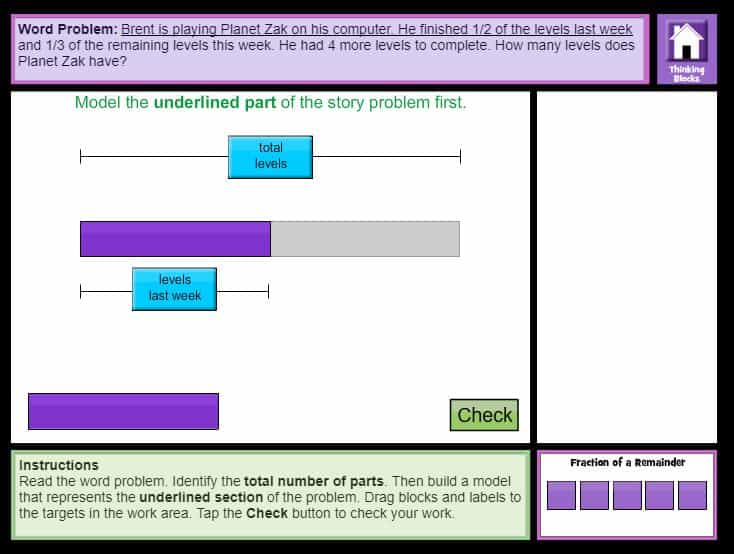# Problem Solving – Interactive Tutorials and Problems Fractions

Thinking Blocks Interactive Tutorials – Model and solve word problems with  fractions.  Online interactive tutorial fourth and fifth grade.

#### Online Word Problems with Fractions Level 2CCSS.MATH.CONTENT.4.NF.B.3.D
Solve word problems involving addition and subtraction of fractions referring to the same whole and having like denominators, e.g., by using visual fraction models and equations to represent the problem.

CCSS.MATH.CONTENT.4.NF.B.4.C
Solve word problems involving multiplication of a fraction by a whole number, e.g., by using visual fraction models and equations to represent the problem.

CCSS.MATH.CONTENT.5.NF.A.2
Solve word problems involving addition and subtraction of fractions referring to the same whole, including cases of unlike denominators, e.g., by using visual fraction models or equations to represent the problem. Use benchmark fractions and number sense of fractions to estimate mentally and assess the reasonableness of answers